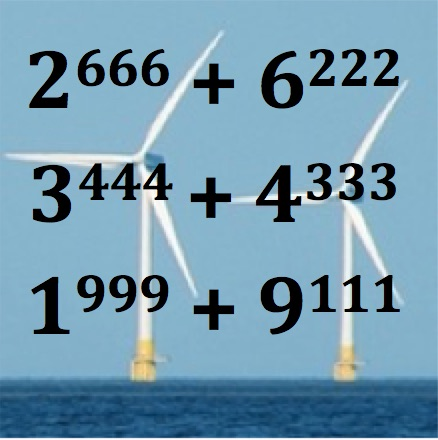Is this number $$3^{444} + 4^{333}$$ divisible by 5?

Hint: Just look at the last digits of powers. What values do they take?

Investigate other big powers?

Make up some similar numerical expressions involving powers that have interesting properties.

What about even bigger powers $$2^{666} + 6^{222}$$

$$7^{888} + 8^{777}$$?